﻿ 基于用户属性与评分相似因子的推荐算法研究 Research on Recommendation Algorithm Based on User’s Attributes and Score Similarity Factors

Computer Science and Application
Vol.08 No.01(2018), Article ID:23459,8 pages
10.12677/CSA.2018.81001

Research on Recommendation Algorithm Based on User’s Attributes and Score Similarity Factors

Peisheng Shi, Jun He, Li Shu, Hao Yin, Junkai Feng

School of Computer, Sichuan University, Chengdu Sichuan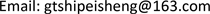Received: Jan. 1st, 2018; accepted: Jan. 12th, 2018; published: Jan. 19th, 2018ABSTRACT

In order to make the user receive more accurate and more personalized recommendation information, this paper improves the influence of the current recommendation system due to the sparse data and the cold start problem. This paper takes the basic attributes of the user, the score timestamp and the user's rating, The similarity factor of the project is combined with the cooperative filtering algorithm, and a cold start recommendation algorithm based on the combination of basic attributes and similarity factors is proposed. This method exhibits better recommendation accuracy and good adaptability to data sparseness by comparing experiments with traditional methods on the Movie Lens dataset.

Keywords:Recommendation Algorithm, Collaborative Filtering, Cold Start, Similarity Factor, User-SimilarityCopyright © 2018 by authors and Hans Publishers Inc.1. 引言

2. 本文算法

$Sim\left(u,v\right)=\lambda Si{m}_{Attr}\left(u,v\right)+\left(1-\lambda \right)Si{m}_{Score}\left(u,v\right)$ (1)

$Si{m}_{Attr}\left(u,v\right)$ 为用户基本属性的相似度， $Si{m}_{Score}\left(u,v\right)$ 为用户评分的相似度； $\lambda$ 为用户的基本属性在相似度计算中所占的权重比例， $\left(1-\lambda \right)$ 为用户对项目的评价占整个相似度计算的权重比例。

$\lambda =\frac{2}{1+\mathrm{exp}\left({I}_{i}\right)}$ (2)

2.1. 用户基本属性相似度

2.1.1. 用户文本型属性相似值

$S\left(k\right)=\left\{\begin{array}{l}1,\text{\hspace{0.17em}}\text{\hspace{0.17em}}{P}_{{u}_{i}}^{\left(k\right)}={P}_{{u}_{j}}^{\left(k\right)}\\ 0,\text{\hspace{0.17em}}\text{\hspace{0.17em}}{P}_{{u}_{i}}^{\left(k\right)}\ne {P}_{{u}_{j}}^{\left(k\right)}\end{array}$ (3)

2.1.2. 用户数值型属性相似值

① 关于年龄Age的分段函数，设Ageu与Agev为用户u、v的年龄，则有相似值SAge为：

${S}_{Age}=\left\{\begin{array}{l}1,\text{\hspace{0.17em}}\text{\hspace{0.17em}}\text{\hspace{0.17em}}\text{\hspace{0.17em}}\text{\hspace{0.17em}}\text{\hspace{0.17em}}\text{\hspace{0.17em}}\text{\hspace{0.17em}}\text{\hspace{0.17em}}\text{\hspace{0.17em}}\text{\hspace{0.17em}}\text{\hspace{0.17em}}\text{\hspace{0.17em}}\text{\hspace{0.17em}}\text{\hspace{0.17em}}\text{\hspace{0.17em}}\text{\hspace{0.17em}}\text{\hspace{0.17em}}\text{\hspace{0.17em}}\text{\hspace{0.17em}}\text{ }\text{\hspace{0.17em}}Ag{e}_{u}=Ag{e}_{v}\\ \frac{1}{|Ag{e}_{u}-Ag{e}_{v}|},\text{\hspace{0.17em}}\text{\hspace{0.17em}}\text{\hspace{0.17em}}Ag{e}_{u}\ne Ag{e}_{v}\end{array}$ (4)

SAge的取值为 $\left\{1,\frac{1}{2},\frac{1}{3},\cdots ,\frac{1}{n}\right\}$

② 关于评分时间分段取值，Tu = tu − ti；tu、ti分别为用户u发布评论时间、项目i生成时间，Tu为两者差值，差值越小证明用户u越活跃。

${S}_{Time}=\left\{\begin{array}{l}1,\text{\hspace{0.17em}}\text{\hspace{0.17em}}\text{\hspace{0.17em}}\text{ }0\le {T}_{u}\le 1\text{\hspace{0.17em}}\text{week};\\ \frac{1}{2},\text{\hspace{0.17em}}\text{\hspace{0.17em}}1\text{\hspace{0.17em}}\text{week}\le {T}_{u}\le 1\text{\hspace{0.17em}}\text{month};\\ \frac{1}{3},\text{\hspace{0.17em}}\text{\hspace{0.17em}}\text{ }1\text{\hspace{0.17em}}\text{month}\le {T}_{u}\le 1\text{\hspace{0.17em}}\text{year};\\ 0,\text{\hspace{0.17em}}\text{\hspace{0.17em}}\text{ }\text{ }{T}_{u}>1\text{\hspace{0.17em}}\text{year}\text{\hspace{0.17em}}\text{or}\text{\hspace{0.17em}}{T}_{u}=0;\end{array}$ (5)

$Si{m}_{Attr}={\sum }_{i\in Attr}{\omega }_{i}×{S}_{Attr}\left(u,v,i\right)$ (6)

${\sum }_{i=1}^{n}{\omega }_{i}=1$ (7)

2.2. 用户评分相似度

2.2.1. 用户评分的相似因子

$Si{m}_{1}\left(u,v\right)=1-\frac{1}{1+\mathrm{exp}\left(-|{R}_{up}-{R}_{vp}|\right)}$ (8)

Rup与Rvp分别表示用户u、v对项目p的评分，若用户u与用户v对于项目之间的评分差越小，则式(8)的值越大，表示用户U与用户V的相似性取值越高， $Si{m}_{1}\left(u,v\right)$ 的取值范围为(0, 1/2)。

2.2.2. 用户偏好的相似因子

$Si{m}_{2}\left(u,v\right)=\frac{1}{1+\mathrm{exp}\left(-\left({R}_{up}-\stackrel{¯}{{R}_{u}}\right)\left({R}_{vp}-\stackrel{¯}{{R}_{v}}\right)\right)}$ (9)

$\left({R}_{up}-\stackrel{¯}{{R}_{u}}\right),\left({R}_{vp}-\stackrel{¯}{{R}_{v}}\right)$ 为一正向偏移、一负向偏移，且均较大偏移值。则 $Si{m}_{2}\left(u,v\right)$ 取值较小，此相似因素较低。

$\left({R}_{up}-\stackrel{¯}{{R}_{u}}\right),\left({R}_{vp}-\stackrel{¯}{{R}_{v}}\right)$ 两正向偏移或者两负向偏移，且有较大偏移值。则 $Si{m}_{2}\left(u,v\right)$ 取值趋近于1，此种情况下相似因素较高。

③ 若 $\left({R}_{up}-\stackrel{¯}{{R}_{u}}\right),\left({R}_{vp}-\stackrel{¯}{{R}_{v}}\right)$ 一正向偏移、一负向偏移，但是偏移值较小，则 $Si{m}_{2}\left(u,v\right)$ 趋近于1/2，则相似因素趋于中间值附近。

2.2.3. 用户评价项目的相似因子

$Si{m}_{3}\left({U}_{i},{U}_{j}\right)=\frac{|{I}_{{U}_{i}}\cap {I}_{{U}_{j}}|}{|{I}_{{U}_{i}}\cup {I}_{{U}_{j}}|}$ (10)

2.2.4. 相似因子综合计算

$Si{m}_{score}\left(u,v\right)=\left(\frac{1}{{I}_{uv}}{\sum }_{i\in {I}_{uv}}Si{m}_{1}\left(u,v,i\right)\cdot Si{m}_{2}\left(u,v,i\right)\right)\cdot Si{m}_{3}\left(u,v\right)$ (11)

2.3. 本文算法设计

Step 1：根据用户基本属性计算出属性相似度矩阵；

Step 2：根据用户历史行为记录计算出评分相似度矩阵；

Step 3：根据用户属性与评分相似度矩阵计算出最终用户相似度矩阵；

Step 4：根据用户最终相似度矩阵得到待测用户u最相似的K个近邻，通过式(12)可以得到相应的预测评分，以获得TOP-N推荐方案。

$\stackrel{¯}{{r}_{ui}}=\frac{{\sum }_{k\in {N}_{K}}Sim\left(u,i\right)\cdot {r}_{ki}}{{\sum }_{k\in {N}_{K}}Sim\left(u,i\right)}$ (12)

NK代表与用户相似度最高的K个邻居；rki表示用户k对项目i的评分。

3. 实验数据展示

3.1. 数据集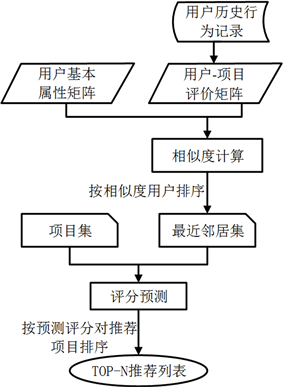Figure 1. Algorithm recommendation flow chart in this paper

3.2. 评价标准

$\text{MAE}=\frac{{\sum }_{i=0}^{N}|\stackrel{¯}{{r}_{ui}}-{r}_{ui}|}{N}$ (13)

rui表示用户u对一系列项目的实际评分；N为项目个数， $\stackrel{¯}{{r}_{ui}}$ 表示为项目的预测分数。

3.3. 实验结果与分析

3.3.1. 预测准确率

3.3.2. 数据的稀疏度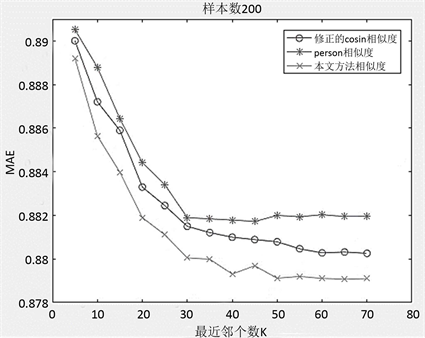Figure 2. The effect comparison of the algorithm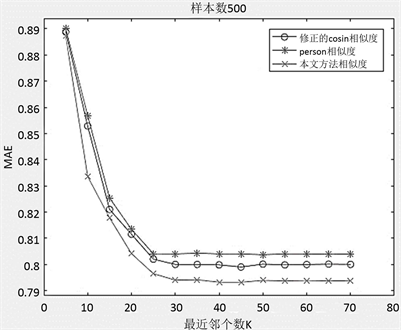Figure 3. The effect comparison of the algorithm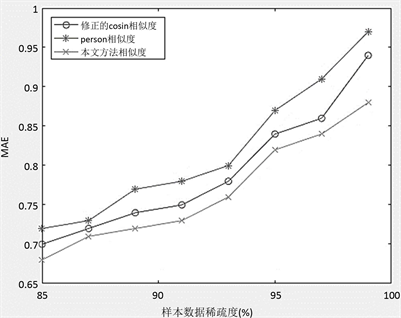Figure 4. MAE of the algorithm

4. 结束语

Research on Recommendation Algorithm Based on User’s Attributes and Score Similarity Factors[J]. 计算机科学与应用, 2018, 08(01): 1-8. http://dx.doi.org/10.12677/CSA.2018.81001

1. 1. Ioannis, K. and Vassilios, S. (2009) On Social Networks and Collaborative Recommendation. Proceedings of the 32nd International ACM SIGIR Conference on Research and Development in Information Retrieval, New York, 195-202.

2. 2. Sarwar, B.M., Konstan, J.A., Borchers, A., et al. (1998) Using Filtering Agents to Improve Prediction Quality in the Grouplens Research Collaborative Filtering System. Proceedings of the 1998 ACM Conference on Computer Supported Cooperative Work, November 14-18 1998, Seattle, 345-354. https://doi.org/10.1145/289444.289509

3. 3. Pazzani, M.J. and Billsus, D. (2007) Content-Based Recommendation Systems. The Adaptive Web, 325-341. https://doi.org/10.1007/978-3-540-72079-9_10

4. 4. Cacheda, F., Carneiro, V., Fernandez, D., et al. (2011) Comparison of Collaborative Filtering Algorithms: Limitations of Current Techniques and Proposals for Scalable, High-Performance Recommender Systems. ACM Transactions on the Web, 5. https://doi.org/10.1145/1921591.1921593

5. 5. Sarwar, B., Karypis, G., Konstan, J., et al. (2001) Item-Based Collaborative Filtering Recommendation Algorithms. Proceedings of the 10th International Conference on World Wide Web, May 1-5 2001, Hong Kong, 285-295. https://doi.org/10.1145/371920.372071

6. 6. Liang, C.Y. and Leng, Y.J. (2014) Collaborative Filtering Based on Infor-mation-Theoretic Co-Clustering. International Journal of Systems Science, 45, 589-597. https://doi.org/10.1080/00207721.2012.724109

7. 7. Bobadilla, J., Serradilla, F. and Bernal, J. (2010) A New Collaborative Filtering Metric That Improves the Behavior of Recommender Systems. Knowledge-Based Systems, 23, 520-528. https://doi.org/10.1016/j.knosys.2010.03.009

8. 8. Reina, D.G., Toral, S.L., Johnson, P., et al. (2014) Improving Discovery Phase of Reactive Ad Hoc Routing Protocols Using Jaccard Distance. The Journal of Super-Computing, 67, 131-152. https://doi.org/10.1007/s11227-013-0992-x

9. 9. Deshpande, M. and Karypis, G. (2004) Item-Based Top-N Recommendation Algorithms. ACM Transactions on Information Systems, 22, 143-177. https://doi.org/10.1145/963770.963776

10. 10. Park, Y.J. and Tuzhilin, A. (2008) The Long Tail of Recommender Systems and How to Leverage It. Proceedings of the 2008 ACM Conference on Recommender Systems, October 23-25 2008, Lausanne, 11-18. |https://doi.org/10.1145/1454008.1454012

11. 11. Martinez, L., Perez, L.G. and Barranco, M.J. (2009) Incomplete Preference Relations to Smooth out the Cold-Start in Collaborative Recommender Systems. 2009 IEEE Annual Meeting of the North American Fuzzy Information Pro- cessing Society (NAFIPS), June 14-17 2009, Cincinnati, 1-6. https://doi.org/10.1109/NAFIPS.2009.5156454

12. 12. Gunawardana, A. and Meek, C. (2008) Tied Boltzmann Machines for Cold Start Recommendations. Proceedings of the 2008 ACM Conference on Recommender Systems, October 23-25 2008, Lausanne, 19-26. https://doi.org/10.1145/1454008.1454013

13. 13. Gunawardana, A. and Meek, C. (2009) A Unified Approach to Building Hybrid Recommender Systems. Proceedings of the 3rd ACM Conference on Recommender Systems, October 23-25 2009, New York, 117-124. https://doi.org/10.1145/1639714.1639735

14. 14. Park, S.T. and Chu, W. (2009) Pairwise Preference Regression Forcold-Start Recommendation. Proceedings of the 2008 ACM Conference on Recommender Systems, October 23-25 2008, Lausanne, 21-28.